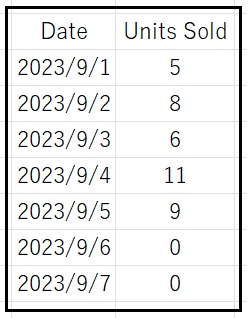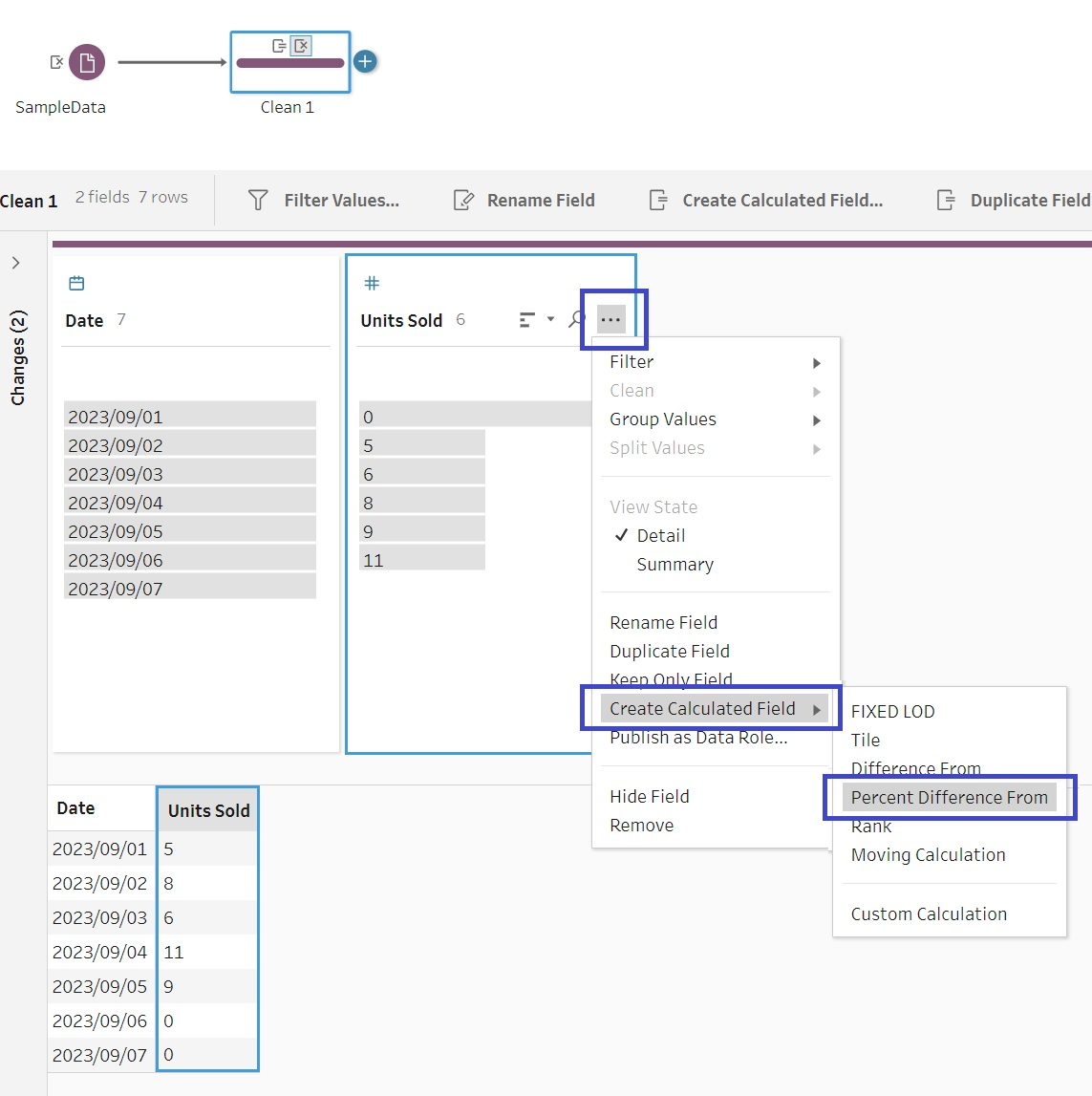(和文はこちらから)

## Introduction:

Tableau Prep Builder, allows limited multirow calculations. This can be helpful during the data preparation step. The details can be found in this post.

## How to Achieve it:

Following calculations are currently possible in Tableau Prep Builder:

#### Difference from:

Computes the difference between the current row value and another value.

#### Percent difference from:

Computes the difference between the current row value and another value as a percentage.

#### Moving calculations:

Returns the sum or average of a numeric field within a flexible set of rows.

Using an example, let us see the steps involved in performing a multirow calculation.Connect the data to Tableau Prep Builder. Add a “Clean Step” to the flow, and here we can add a new field (eg. Percent difference from previous value) based on multi row level calculation as shown below.A popup window will appear as shown below, here users can define the nature of the calculation. Finally click “Done” button to save the new calculation and continue data processing.## Summary:

Tableau Prep Builder started supporting multirow calculations, this can be of some help during the data processing step.